0708-1300/Class notes for Thursday, October 18

(diff) ← Older revision | Latest revision (diff) | Newer revision → (diff)
Announcements go here

Class Notes

The notes below are by the students and for the students. Hopefully they are useful, but they come with no guarantee of any kind.

Outline

Today we stated the Whitney Embedding Theorem and began to discuss its proof. Along the way, we also encountered some related notions that will serve us well in the future. We begin by stating the theorem:

Theorem (Whitney Embedding)

Let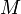$M\!$ be a smooth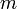$m\!$-manifold. Then there exists an embedding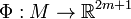$\Phi : M \to \mathbb{R}^{2m+1}$.

Proof

Outline

We will break the proof of the theorem into three parts:

1. Find an embedding of a compact$M\!$ into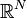$\mathbb{R}^N\!$ for some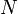$N\!$.
2. Use Sard's Theorem to reduce$N\!$ to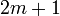$2m+1\!$.
3. Use the "Zebra Trick" to prove the theorem for non-compact$M\!$.

Parts two and three shall be left to the next lecture.

Part 1

Suppose that$M\!$ is compact. Let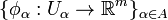$\{\phi_\alpha : U_\alpha \to \mathbb{R}^m \}_{\alpha \in A}$ be an atlas for$M\!$, and note that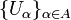$\{U_\alpha\}_{\alpha \in A}\!$ is an open cover of$M\!$. Hence it possesses a finite subcover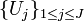$\{U_j\}_{1 \le j \le J}\!$, and the corresponding collection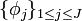$\{\phi_j\}_{1 \le j \le J}$ of charts is an atlas.

Choose smooth functions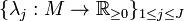$\{\lambda_j : M \to \mathbb{R}_{\ge 0} \}_{1 \le j \le J}$ with the following properties:

1.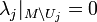$\lambda_j |_{M \setminus U_j} = 0$
2.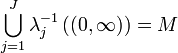$\bigcup_{j=1}^{J} \lambda_j^{-1}\left((0,\infty)\right) = M$
3.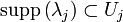$\mathrm{supp}\left(\lambda_j\right) \subset U_j$ for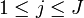$1 \le j \le J$

where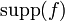$\mathrm{supp}(f)\!$ for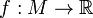$f : M \to \mathbb{R}$ is the support of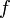$f\!$, ie. the closure of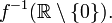$f^{-1}(\mathbb{R} \setminus \{0\}).$ The existence of such functions follows from the existence of smooth partitions of unity for manifolds---a concept that will be discussed later on.

Now define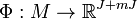$\Phi : M \to \mathbb{R}^{J + mJ}$ by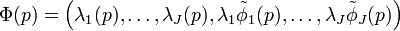$\Phi(p) = \left(\lambda_1(p), \ldots, \lambda_J(p), \lambda_1 \tilde \phi_1 (p), \ldots, \lambda_J \tilde \phi_J(p)\right)$, where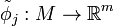$\tilde \phi_j : M \to \mathbb{R}^m$ is defined by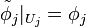$\tilde \phi_j |_{U_j} = \phi_j$ and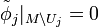$\tilde \phi_j |_{M \setminus U_j} = 0$.

We claim that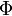$\Phi\!$ is an embedding. That$\Phi\!$ is smooth follows immediately from its construction (the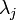$\lambda_j\!$s have been used to smear out the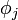$\phi_j\!$ to smooth functions on all of$M\!$). That$\Phi\!$ is injective is also clear. It takes a bit of work to show that$\Phi\!$ is an immersion, but this is left as an exercise. It remains to see that$\Phi\!$ is a homeomorphism, but this fact follows from the following topological lemma.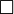$\Box\!$

Lemma

Let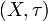$(X,\tau)\!$ and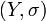$(Y,\sigma)\!$ be topological spaces. Suppose that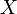$X\!$ is compact,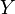$Y\!$ is Hausdorff, and that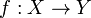$f : X \to Y$ is continuous and injective. Then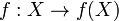$f : X \to f(X)$ is a homeomorphism.

Proof

Since$f\!$ is an injection onto its image, it is a bijection. Since$f\!$ is continuous, it remains to show that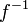$f^{-1}\!$ is continuous. Thus, it suffices to see that$f\!$ takes closed sets to closed sets. Let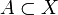$A \subset X$ be closed. Since$X\!$ is compact, so is$A\!$. Hence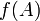$f(A)\!$ is compact since$f\!$ is continuous. But$Y\!$ is Hausdorff, and every compact subset of a Hausdorff space is closed. Hence$f(A)\!$ is closed.$\Box$

Remarks

The smearing functions we used in Part 1 of the proof of the Whitney Embedding Theorem are very similar to partitions of unity---collections of functions that break the constant function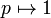$p \mapsto 1$ into a bunch of bump functions. We will now formalize this notion and show that such collections of functions exist for smooth manifolds.

Definition

Let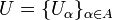$U = \{U_\alpha\}_{\alpha \in A}$ be an open cover of a topological space (manifold)$M\!$. A partition of unity subordinate to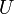$U\!$ is a collection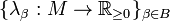$\{\lambda_\beta : M \to \mathbb{R}_{\ge 0} \}_{\beta \in B}$ of continuous (smooth) functions such that

1. For every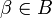$\beta \in B$ there is an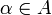$\alpha \in A$ such that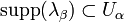$\mathrm{supp}(\lambda_\beta) \subset U_\alpha$
2.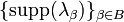$\{\mathrm{supp}(\lambda_\beta)\}_{\beta \in B}$ is locally finite, ie. for every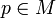$p \in M$, there exists a neighbourhood$U$ of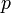$p$ such that$U$ intersects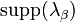$\mathrm{supp}(\lambda_\beta)$ for only finitely many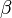$\beta\!$.
3.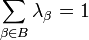$\sum_{\beta \in B} \lambda_\beta = 1$

A local refinement of$U\!$ is an open cover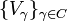$\{V_\gamma\}_{\gamma \in C}$ of$M\!$ such that for every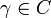$\gamma \in C$,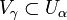$V_\gamma \subset U_\alpha$ for some$\alpha \in A$.$M\!$ is called paracompact if every open cover of$M\!$ has a locally finite refinement.

Remarks

For further information on paracompactness, we refer the reader to the corresponding Wikipedia entry. Note, in particular, that locally compact, second-countable topological spaces---such as manifolds---are paracompact, and that paracompact spaces are shrinking spaces. The following result (which follows immediately from these facts) will be useful for constructing partitions of unity on manifolds:

Theorem

Manifolds are paracompact. In particular, if$\{U_\alpha\}_{\alpha \in A}$ is locally finite then there is an open cover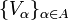$\{V_\alpha\}_{\alpha \in A}$ such that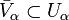$\bar{V}_\alpha \subset U_\alpha$ for every$\alpha \in A$.